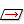# Dip Vector Plots

A Dip Vector directly represents the maximum dip of a plane and is orthogonal (90 degrees) to the pole vector of a plane. A Dip Vector is located at the midpoint of the great circle projection of a given plane. To view a Dip Vector plot:

• The Pole Vector Mode / Dip Vector Mode must be set to Dip Vectormode
• Choose the Vector Plotoption from the toolbar or the View menu, OR
• In the Sidebar choose the Dip Vector Display checkbox and the Dip radio button.

A Dip Vector plot is an alternative to the Pole Plot representation of planar data on a stereonet. Dip vectors have a one-to-one correspondence with pole vectors (i.e., for each plane there is one pole vector and one dip vector so the number of pole vectors or dip vectors are equal for a given file).

## Kinematic Analysis

Either pole vectors or dip vectors can be used for Kinematic Analysis. Dip vectors are preferred by some users for various Kinematic Analysis methods, and so dip vector plotting is available for applicable Kinematic Analysis options (Planar Sliding and Flexural Toppling).

## Sets and Dip Vectors

It is important to note that in general the creation of joint sets from Dip Vectors is not valid, and therefore all Set options are disabled in Dip Vector Mode. If you wish to define joint sets and the Set options are disabled, then you must switch to Pole Vector Mode first, you will then be able to define Sets.

## Contouring Dip Vectors

Dip vector plots can be contoured just like pole plots. If you are in Dip Vector mode the Contour Plot will automatically contour the dip vectors.

## Overlay Plot Types

The Sidebar plotting options allow you to overlay dip vectors, contours, planes, intersections, etc. See the Overlay Plots topic for details.